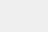# 一文上手最新TensorFlow2.0系列（二）

### 本文是全系列中第19 / 23篇：TensorFlow 从入门到精通【磐创AI导读】：本系列文章介绍了与tensorflow的相关知识，包括其介绍、安装及使用等。本篇文章将接着上篇文章继续介绍它的安装及部分使用。查看上篇：文末福利｜一文上手TensorFlow2.0（一）要获取更多的机器学习、深度学习资源，欢迎大家点击上方蓝字关注我们的公众号：磐创AI

• Tensorflow2.0 介绍
• Tensorflow 常见基本概念
• 从1.x 到2.0 的变化
• Tensorflow2.0 的架构
• Tensorflow2.0 的安装(CPU和GPU)
• Tensorflow2.0 使用
• “tf.data” API
• ### “tf.keras”API

• 使用GPU加速
• 安装配置GPU环境
• 使用Tensorflow-GPU

## 2. TensorFlow2.0安装

Tensorflow兼容性最好的是Unix内核的系统，如Linux，MacOS等。另外TensorFlow的GPU版本仅支持Linux环境，不支持Windows和Mac环境，因此本节的安装部分仅针对Linux系统环境。我们会统一使用Anaconda，在Mac和Windows下安装的过程也较为简单，读者可以自行参考其官方文档。Anaconda官网有各个平台详细的安装使用教程：https://docs.anaconda.com/anaconda/install/。1. 安装Anaconda

（1）安装Anaconda3.提示是否要写入配置文件，输入“yes”回车。4.提示是否安装“VSCode”，输入“no”回车。安装完成。（2）创建虚拟环境

``conda create --name apython python=3.6``

``alias python="/usr/bin/python2"alias python3="/usr/bin/python3"alias apython="/home/lqhou/anaconda3/envs/apython/bin/python3"alias apip="/home/lqhou/anaconda3/envs/apython/bin/pip"``2. 安装TensorFlow
GPU版的TensorFlow包含了CPU版本，如果读者手上有GPU资源的话，可以直接参考后文会提到的安装GPU版的TensorFlow。``apip install tensorflow-2.0.0a0-cp36-cp36m-manylinux1_x86_64.whl``3. 使用Jupyter Notebook
），后面章节的项目和代码，读者可以任意选择自己喜欢的编程工具。## 3.1 “tf.data”API

• Extract：从硬盘中读取数据（可以是本地的也可以是云端的）。

• Transform：数据的预处理（例如数据清洗、格式转换等）。``import tensorflow as tfimport pathlib``
pathlib提供了一组用于处理文件系统路径的类。导入需要的包后，可以先检查一下TensorFlow的版本：
``print(tf.__version__)``

``# 获取当前路径data_root = pathlib.Path.cwd()# 获取指定目录下的文件路径（返回是一个列表，每一个元素是一个PosixPath对象）all_image_paths = list(data_root.glob('*/*/*'))print(type(all_image_paths))# 将PosixPath对象转为字符串all_image_paths = [str(path) for path in all_image_paths]print(all_image_paths)print(data_root)````# 获取图片类别的名称，即存放样本图片的五个文件夹的名称label_names = sorted(item.name for item in data_root.glob('*/*/') if item.is_dir())# 将类别名称转为数值型的类标label_to_index = dict((name, index) for index, name in enumerate(label_names))# 获取所有图片的类标all_image_labels = [label_to_index[pathlib.Path(path).parent.name]print(label_to_index)print("First 10 labels indices: ", all_image_labels[:2])print("First 10 labels indices: ", all_image_paths[:2])````def load_and_preprocess_image(path): # 读取图片 image = tf.io.read_file(path) # 将jpeg格式的图片解码，得到一个张量（三维的矩阵） image = tf.image.decode_jpeg(image, channels=3) # 由于数据集中每张图片的大小不一样，统一调整为192*192 image = tf.image.resize(image, [192, 192]) # 对每个像素点的RGB值做归一化处理 image /= 255.0  return image``

``# 构建图片路径的“dataset”path_ds = tf.data.Dataset.from_tensor_slices(all_image_paths)# 使用AUTOTUNE自动调节管道参数AUTOTUNE = tf.data.experimental.AUTOTUNE# 构建图片数据的“dataset”image_ds = path_ds.map(load_and_preprocess_image,num_parallel_calls=AUTOTUNE)# 构建类标数据的“dataset”label_ds = tf.data.Dataset.from_tensor_slices(tf.cast(all_image_labels, tf.int64))# 将图片和类标压缩为（图片，类标）对image_label_ds = tf.data.Dataset.zip((image_ds, label_ds))print(image_ds)print(label_ds)print(image_label_ds)````import matplotlib.pyplot as pltplt.figure(figsize=(8,8))for n,image_label in enumerate(image_label_ds.take(4)): plt.subplot(2,2,n+1) plt.imshow(image_label) plt.grid(False) plt.xticks([]) plt.yticks([]) plt.xlabel(image_label)````# 下载的模型在用户根目录下，具体位置：“~/.keras/models/mobilenet_v2_weights_tf_dim_ordering_tf_kernels_1.0_192_no_top.h5”mobile_net = tf.keras.applications.MobileNetV2(input_shape=(192, 192, 3),include_top=False)# 禁止训练更新“MobileNetV2”模型的参数mobile_net.trainable = False``

``# 使用Dataset类的shuffle方法打乱数据集image_count = len(all_image_paths)ds = image_label_ds.shuffle(buffer_size=image_count)# 让数据集重复多次ds = ds.repeat()# 设置每个batch的大小BATCH_SIZE = 32ds = ds.batch(BATCH_SIZE)# 通过“prefetch”方法让模型的训练和每个batch数据集的加载并行ds = ds.prefetch(buffer_size=AUTOTUNE)``

``# 定义一个函数用来将范围在[0,1]之间的数据映射到[-1,1]之间def change_range(image,label): return 2*image-1, label# 使用“map”方法对dataset进行处理keras_ds = ds.map(change_range)``

``model = tf.keras.Sequential([ mobile_net, tf.keras.layers.GlobalAveragePooling2D(), tf.keras.layers.Dense(len(label_names))])``

``model.compile(optimizer=tf.keras.optimizers.Adam(), loss='sparse_categorical_crossentropy', metrics=["accuracy"])model.summary()``
“model.summary()”可以输出模型各层的参数概况，如图19所示：``model.fit(ds, epochs=1, steps_per_epoch=10)``/  今日赠送书籍 /《TensorFlow与卷积神经网络》/  今日留言主题 /● 常用的聚类算法及聚类算法评价指标
● 七大Github机器学习热门项目
● 机器学习在生活中的九大有趣应用点击下方 |  | 了解更多